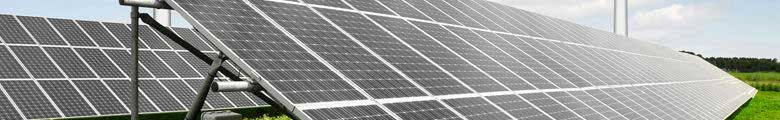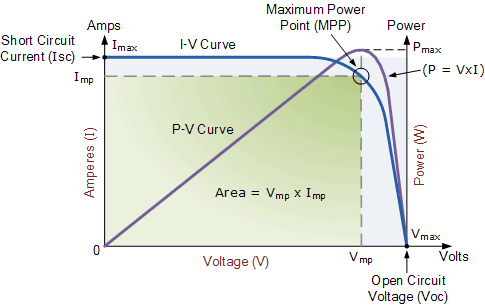# Solar Cell I-V Characteristic

## Solar Cell I-V Characteristic and the Solar Cell I-V CurveSolar Cell I-V Characteristic Curves show the current and voltage ( I-V ) characteristics of a particular photovoltaic ( PV ) cell, module or array giving a detailed description of its solar energy conversion ability and efficiency. Knowing the electrical I-V characteristics (more importantly Pmax) of a solar cell, or panel is critical in determining the device’s output performance and solar efficiency.

Photovoltaic solar cells convert the suns radiant light directly into electricity. With increasing demand for a clean energy source and the sun’s potential as a free energy source, has made solar energy conversion as part of a mixture of renewable energy sources increasingly important. As a result, the demand for efficient solar cells, which convert sunlight directly into electricity, is growing faster than ever before.Photovoltaic ( PV ) cells are made made almost entirely from silicon that has been processed into an extremely pure crystalline form that absorbs the photons from sunlight and then releases them as electrons, causing an electric current to flow when the photoconductive cell is connected to an external load. There are a variety of different measurements we can make to determine the solar cell’s performance, such as its power output and its conversion efficiency.

The main electrical characteristics of a PV cell or module are summarized in the relationship between the current and voltage produced on a typical solar cell I-V characteristics curve. The intensity of the solar radiation (insolation) that hits the cell controls the current ( I ), while the increases in the temperature of the solar cell reduces its voltage ( V ).

Solar cells produce direct current ( DC ) electricity and current times voltage equals power, so we can create solar cell I-V curves representing the current versus the voltage for a photovoltaic device.

Solar Cell I-V Characteristics Curves are basically a graphical representation of the operation of a solar cell or module summarising the relationship between the current and voltage at the existing conditions of irradiance and temperature. I-V curves provide the information required to configure a solar system so that it can operate as close to its optimal peak power point (MPP) as possible.

### Solar Cell I-V Characteristic CurveThe above graph shows the current-voltage ( I-V ) characteristics of a typical silicon PV cell operating under normal conditions. The power delivered by a solar cell is the product of current and voltage ( I x V ). If the multiplication is done, point for point, for all voltages from short-circuit to open-circuit conditions, the power curve above is obtained for a given radiation level.

With the solar cell open-circuited, that is not connected to any load, the current will be at its minimum (zero) and the voltage across the cell is at its maximum, known as the solar cells open circuit voltage, or Voc. At the other extreme, when the solar cell is short circuited, that is the positive and negative leads connected together, the voltage across the cell is at its minimum (zero) but the current flowing out of the cell reaches its maximum, known as the solar cells short circuit current, or Isc.

Then the span of the solar cell I-V characteristics curve ranges from the short circuit current ( Isc ) at zero output volts, to zero current at the full open circuit voltage ( Voc ). In other words, the maximum voltage available from a cell is at open circuit, and the maximum current at closed circuit. Of course, neither of these two conditions generates any electrical power, but there must be a point somewhere in between were the solar cell generates maximum power.

However, there is one particular combination of current and voltage for which the power reaches its maximum value, at Imp and Vmp. In other words, the point at which the cell generates maximum electrical power and this is shown at the top right area of the green rectangle. This is the “maximum power point” or MPP. Therefore the ideal operation of a photovoltaic cell (or panel) is defined to be at the maximum power point.

The maximum power point (MPP) of a solar cell is positioned near the bend in the I-V characteristics curve. The corresponding values of Vmp and Imp can be estimated from the open circuit voltage and the short circuit current: Vmp ≅ (0.8–0.90)Voc and Imp ≅ (0.85–0.95)Isc. Since solar cell output voltage and current both depend on temperature, the actual output power will vary with changes in ambient temperature.

Thus far we have looked at Solar Cell I-V Characteristic Curve for a single solar cell or panel. But many photovoltaic arrays are made up of smaller PV panels connected together. Then the I-V curve of a PV array is just a scaled up version of the single solar cell I-V characteristic curve as shown.

### Solar Panel I-V Characteristic CurvesPhotovoltaic panels can be wired or connected together in either series or parallel combinations, or both to increase the voltage or current capacity of the solar array. If the array panels are connected together in a series combination, then the voltage increases and if connected together in parallel then the current increases. The electrical power in Watts, generated by these different photovoltaic combinations will still be the product of the voltage times the current, ( P = V x I ). However the solar panels are connected together, the upper right hand corner will always be the maximum power point (MPP) of the array.

## The Electrical Characteristics of a Photovoltaic Array

The electrical characteristics of a photovoltaic array are summarised in the relationship between the output current and voltage. The amount and intensity of solar insolation (solar irradiance) controls the amount of output current ( I ), and the operating temperature of the solar cells affects the output voltage ( V ) of the PV array. Solar cell I-V characteristic curves that summarise the relationship between the current and voltage are generally provided by the panels manufacturer and are given as:

### Solar Array Parameters

• • VOC = open-circuit voltage: – This is the maximum voltage that the array provides when the terminals are not connected to any load (an open circuit condition). This value is much higher than Vmp which relates to the operation of the PV array which is fixed by the load. This value depends upon the number of PV panels connected together in series.
• • ISC = short-circuit current – The maximum current provided by the PV array when the output connectors are shorted together (a short circuit condition). This value is much higher than Imp which relates to the normal operating circuit current.
• • MPP = maximum power point – This relates to the point where the power supplied by the array that is connected to the load (batteries, inverters) is at its maximum value, where MPP = Imp x Vmp. The maximum power point of a photovoltaic array is measured in Watts (W) or peak Watts (Wp).
• • FF = fill factor – The fill factor is the relationship between the maximum power that the array can actually provide under normal operating conditions and the product of the open-circuit voltage times the short-circuit current, ( Voc x Isc ) This fill factor value gives an idea of the quality of the array and the closer the fill factor is to 1 (unity), the more power the array can provide. Typical values are between 0.7 and 0.8.
• • %eff = percent efficiency – The efficiency of a photovoltaic array is the ratio between the maximum electrical power that the array can produce compared to the amount of solar irradiance hitting the array. The efficiency of a typical solar array is normally low at around 10-12%, depending on the type of cells (monocrystalline, polycrystalline, amorphous or thin film) being used.

Solar Cell I-V Characteristic Curves are graphs of output voltage versus current for different levels of insolation and temperature and can tell you a lot about a PV cell or panel’s ability to convert sunlight into electricity. The most important values for calculating a particular panels power rating are the voltage and current at maximum power.

Some solar panels are rated at slightly higher or lower voltages than others of the same wattage value, and this affects the amount of current available and therefore the panels MPP. Other parameters also important are the open circuit voltage and short circuit current ratings from a safety point of view, especially the voltage rating. An array of six panels in series, while having a nominal 72 volt (6 x 12) rating, could potentially produce an open-circuit voltage of over 120 volts DC, which is more than enough to be dangerous.

Photovoltaic I-V characteristics curves provide the information needed for us to configure a solar power array so that it can operate as close as possible to its maximum peak power point. The peak power point is measured as the PV module produces its maximum amount of power when exposed to solar radiation equivalent to 1000 watts per square metre, 1000 W/m2 or 1kW/m2.

Tagged with:

###### 11 Comments » for Solar Cell I-V Characteristic
1.jeff paul says:

Where is the negative voltage curve, which one might expect for a partially shaded cell in a series string?

2.Nora Dawn says:

The potential of solar energy is almost beyond anyone`s imagination. How can we tap into this vast resource?

•Saurabh says:

by holding a very large cane (as long as the distance between earth and sun) towards the sun and poking the sun with it.

3.Anil Garde says:

What is the difference in Solar “Irradiation” and Solar “Insolation” ?

4.Cuthbert says:

What is the effect of ideal and non ideal recombination on I-V curves

5.lakhan says:

In this experiment we are used cells are connected in series .why?and can we are used in parallel….

•Administrator says:

Connecting solar cells together in series produces a higher voltage for a given current, and connecting solar cells in parallel produces a higher current for a given voltage.

6.HARIOM says:

•Administrator says:
•Dulip Tharaka Madurasinghe says:
•Administrator says: